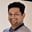Related Tags

java

# What is the stream.collect() method in Java?Tarun Telang

### Overview

The stream.collect() method is used to perform a mutable reduction operation on the elements of a stream. It returns a new mutable object containing the results of the reduction operation.

This method can be used to perform several different types of reduction operations, such as:

• Computing the sum of numeric values in a stream.
• Finding the minimum or maximum value in a stream.
• Constructing a new String by concatenating the contents of a stream.
• Collecting elements into a new List or Set.

### Syntax

The syntax of the stream.collect() method is as follows:

<R, A> R stream.collect(Collector<? super T,A,R> collector)
Syntax of the stream.collect() method

### Parameters

• R: This is the type of the result object.
• T: This is the type of the source element.
• A : This is the type of mutable object used for accumulating results (which is also the type of the result object).
• collector: This is the type of collector that performs the reduction operation.

### Return value

This method returns the result of the reduction operation.

### Example

The following example shows us how to use the Stream.collect() method:

import java.util.*;
import java.util.stream.*;
import static java.util.stream.Collectors.*;

public class CollectExample {
public static void main(String[] args) {
Integer[] intArray = {1, 2, 3, 4, 5};

// Creating a List from an array of elements
// using Arrays.asList() method
List<Integer> list = Arrays.asList(intArray);

// Collecting all elements of the list into a new
// list using collect() method
List<Integer> evenNumbersList = list.stream()
.filter(i -> i%2 == 0)
.collect(toList());
System.out.println(evenNumbersList);

// finding the sum of all the values
// in the stream
Integer sum = list.stream()
.collect(summingInt(i -> i));
System.out.println(sum);

// finding the maximum of all the values
// in the stream
Integer max = list.stream()
.collect(maxBy(Integer::compare)).get();
System.out.println(max);

// finding the minimum of all the values
// in the stream
Integer min = list.stream()
.collect(minBy(Integer::compare)).get();
System.out.println(min);

// counting the values in the stream
Long count = list.stream()
.collect(counting());
System.out.println(count);
}
}


Stream.collect() code example for even numbers

### Explanation

• Line 7: We have a stream of integers, which are the elements of intArray.
• Line 11: We use the Arrays.asList() method to get a List from an array of elements.
• Lines 15–17: We use the stream() method to get a stream from the list. We filter the even elements and collect them into a new list using the collect() method.
• Line 18: We use the System.out.println() method to print the filtered even numbers.
• Lines 22–23: We use the collect() method summingInt(ToIntFunction) as an argument. The summingInt() method returns a collector that sums the integer values extracted from the stream elements by applying an int producing mapping function to each element.
• Line 24: We use the System.out.println() method to print the sum of elements in the stream.
• Lines 28–29: We use the collect() method with maxBy(Comparator) as an argument. The maxBy() accepts a Comparator and returns a collector that extracts the maximum element from the stream according to the given Comparator.
• Line 30: We use the System.out.println() method to print the maximum value in the stream.
• Lines 34–35: We use the collect() method with minBy(Comparator) as an argument. The minBy() accepts a Comparator and returns a collector that extracts the minimum element from the stream according to the given Comparator.
• Lines 39–40: We use the collect() method with counting() as an argument, which returns a collector that counts the number of input elements.
• Line 41: We use the System.out.println() method to print the number of elements in the stream.

RELATED TAGS

java

CONTRIBUTORTarun Telang
RELATED COURSES

View all Courses

Keep Exploring

Learn in-demand tech skills in half the time